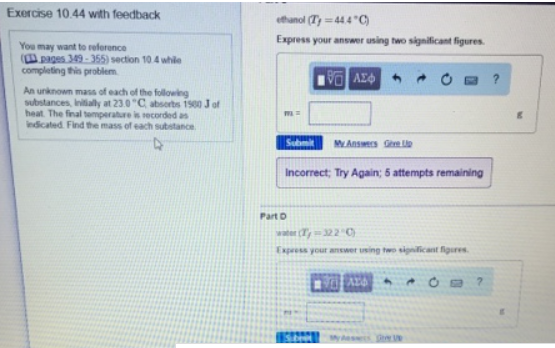# Problem: An unknown mass of each of the following substances, initially at 23.0°C absorbs 1560 J of heat. The final temperature is recorded as indicated. Find the mass of each substance.Part Cethanol (Tf = 44.4°C)Express your answer using two significant figuresPart Cwater (Tf = 32.2°C)Express your answer using two significant figures

###### FREE Expert Solution
83% (185 ratings)###### Problem Details

An unknown mass of each of the following substances, initially at 23.0°C absorbs 1560 J of heat. The final temperature is recorded as indicated. Find the mass of each substance.

Part C

ethanol (Tf = 44.4°C)

Part C

water (Tf = 32.2°C)